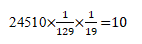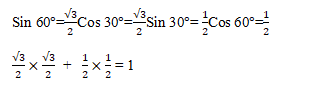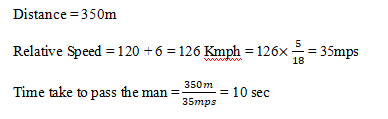# RRB ALP 2018 Practice Test Papers | Arithmetic Questions (Day-45)

Dear Aspirants, Here we have given the Important RRB ALP & Technicians Exam 2018 Practice Test Papers. Candidates those who are preparing for RRB ALP 2018 can practice these Arithmetic Questions to get more confidence to Crack RRB 2018 Examination.

[WpProQuiz 2581]

Click “Start Quiz” to attend these Questions and view Explanation

1. Arvind can do a piece of work in 15 days. Gokul can do a piece of work in 30 days. The whole work completed by both of them in how many days?
1. 5 days
2. 10 days
3. 12 days
4. 15 days
1. The value of 350% of 700 + 500% of 450 = ?
1. 4900
2. 4800
3. 4700
4. 4600
1. Find the value of 24510÷129÷19
1. 12
2. 10
3. 13
4. 14
1. A travel bag with measures 40 cm × 24 cm × 12 cm is to be covered with a tarpaulin cloth. How manymetres of tarpaulin of width 90 cm is required to cover 100 such travel bags?
1. 42.30 m
2. 32.80 m
3. 32.40 m
4. 18.90 m
1. Find the value of

Sin 60°cos 30°+ Sin 30°cos 60°

1. 1
2. -1
3. 0
4. 2
1. Present ages of Akash and Baskar are in the ratio of 5 : 4 respectively. Three years hence, the ratio of their ages will become 11 : 9 respectively. What is Baskar’s present age in years?
1. 24
2. 27
3. 40
4. 48
1. A train of length 350meter is running at a speed of 120 kmph. In what time, it will pass a man who is running at 6kmph in the direction opposite to that in which the train is going?
1. 6 sec
2. 10 sec
3. 12 sec
4. 8 sec
1. The least number which is to be added to make 7399 a perfect square is
1. 170
2. 151
3. 27
4. 0
1. What is the median of the following data?

1, 9, 8, 6, 10, 15, 17

1. 1
2. 9
3. 8
4. 15
1. If each of the dimensions of a rectangle is increased by 200%, then the area is increased by :
1. 300%
2. 200%
3. 800%
4. 600%

Per day work of Arvind = 1/15

Per day work of Gokul = 1/30

Per day work of Arvind + Gokul= 1/15 + 1/30 = 3/30 = 1/10

The whole work completed in 10 days

350/100*700= 2450

500/100* 450= 2250

2450+2250 = 4700Surface Area of Cuboid = 2(lb + lh + bh)

= 2 (40 x 24 + 40 x 12 + 24 x 12)

= 2(960 + 480 + 288)

= 2 x 1458 =  2916

Hence, Surface Area of 100 suitcases = 291600 sqcms

Required tarpaulin will have same area = Area/ Width

= 291600/ 90 = 3240 cm=3240/100 m=32.40 mLet the ages of Akash and Baskar be5x and 4x respectively.

(5x+3)/(4x+3)=11/9

X=67569 is a square value of 87

7569- 7399 = 170

170 is the least number is to added to make 7399 a perfect square.

1, 9, 8, 6, 10, 15, 17

1,6,8,9,10,15,17

Median = 9

Area of rectangle = L × B = LB

Increasing 200% means length and breadth of rectangle becomes three times.

New area of rectangle = 3L × 3B = 9 LB

Thus, area is increased by 800%.

RRB ALP 2018 – “All in One” Study Materials and Practice Sets